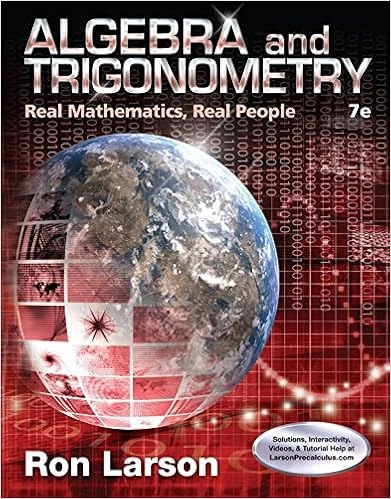# Solutions3 - MATH 2007B Test 3 Solutions November 7...

• Notes
• 2
• 100% (2) 2 out of 2 people found this document helpful

This preview shows page 1 - 2 out of 2 pages.

##### We have textbook solutions for you!
The document you are viewing contains questions related to this textbook.The document you are viewing contains questions related to this textbook.
Chapter 10 / Exercise 13
Algebra and Trigonometry: Real Mathematics, Real People
LarsonExpert Verified
MATH 2007BTest 3 SolutionsNovember 7, 2011[Marks]1. Consider the parametric curvex=t21, y= 2t3+t2.2ydx2.
(b) Determine the point(s) where the tangent is horizontal/vertical.
(c) Determine the intervals oftwhere the graph is concave up/down.
2. Find the length of the parametric curvex= 3t2, y= 2t3,3t8.
##### We have textbook solutions for you!
The document you are viewing contains questions related to this textbook.The document you are viewing contains questions related to this textbook.
Chapter 10 / Exercise 13
Algebra and Trigonometry: Real Mathematics, Real People
LarsonExpert Verified
•••